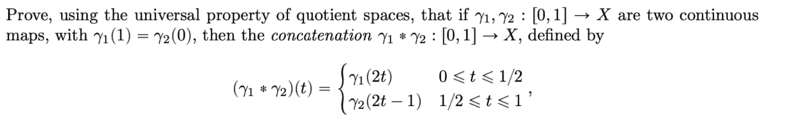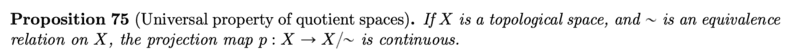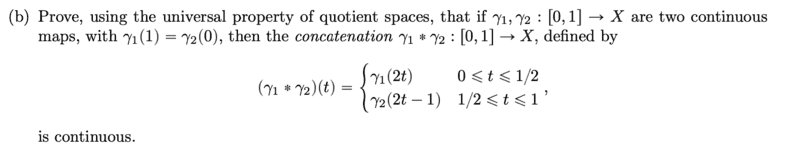# Prove that the concatenation function is continuous

• docnet
In summary, the problem asks to prove the continuity of the function (f*g) with f and g being continuous functions in [0,1] and [1,2] respectively, and f(1)=g(1). To prove this, it is necessary to use the universal property of quotient spaces. However, the solution provided is not correct as it does not define the equivalence relation on the correct set. The correct approach would be to use the standard epsilon-delta definition of continuity for each case of x in [0,2].f

#### docnet

Homework Statement
prove the continuity of the function
Relevant Equations
f,g
Let f be continuous in [0,1] and g be continuous in [1,2] and f(1)=g(1). prove that

$$(f*g)= \begin{cases} f(t), 0\leq t\leq 1\\ g(t), 1\leq t \leq2 \end{cases}$$
is continuous using the universal property of quotient spaces.

Let ##f:[0,1]→X## and ##g:[1,2]→Y##
f and y are continuous, thus for open sets in [0,1] and open sets in [1,2], their images are also open in X and Y, hence X and Y are topological spaces.
f(1)∈X is an element in X and g(1)∈Y is an element in Y.
let W be a discrete union of X and Y, which itself is a topological space
##f(1)=g(1)## is an equivalence relation on W.
Then (f*g) is a projection map from W onto W/(f(1) ~g(1)) and hence continuous by the universal property of quotient spaces

Last edited:
Homework Statement:: prove the continuity of the function
Relevant Equations:: f,g

Let f be continuous in [0,1] and g be continuous in [1,2] and f(1)=g(1). prove that

$$(f*g)= \begin{cases} f(t), 0\leq t\leq 1\ g(t), 1\leq t \leq2 \end{cases}$$
is continuous using the universal property of quotient spaces.

Let f:[0,1]→X and g:[1,2]→Y
f and y are continuous, thus ...
Thus is wrong here. A function is continuous if the pre-image of an open set is open. That is: ##f## is continuous if ##f^{-1}(U) \subseteq [0,1]## is always open when ##U\subseteq X## is open.

... for open sets in [0,1] and open sets in [1,2], their images are also open in X and Y, hence X and Y are topological spaces.

This would be the definition of an open function, i.e. a function that maps open sets to open sets. Open functions do not need to be continuous and continuous functions do not need to be open.

f(1)∈X is an element in X and g(1)∈Y is an element in Y.
let W be a discrete union of X and Y.
What does discrete here mean? You can either consider ##X\cup Y## as subspaces of ##\mathbb{R}## or ##X\times Y## as direct product of two topological spaces. I assume you meant the latter since the rest of the vocabulary fits better on a direct product than a simple union.
f(1)∈X=g(1)∈Y is an equivalence relation on W.
A relation ##R## is a subset of a direct (Cartesian) product of two sets. In this case, I assume you meant a subset of ##W=X\times Y##. An equivalence relation is a special relation. You defined ##R=\{(f(1),g(1))\}## which is only a single point. This is trivially an equivalence relation, but useless, too.

Maybe you meant ##R=\{(x,y)\in W\,|\,\exists \,t\in [0,1] \,\exists \,s\in [1,2]\, : \,x=f(t)\,\wedge\,y=f(s)\,\wedge\,f(1)=g(1)\}## but this is a wild guess.

Another possibility is to consider all such functions ##f,g##. Then ##\mathcal{W}:=\{f\,|\,f:[0,1]\longrightarrow X\} \times \{g\,|\,g:[1,2]\longrightarrow Y\}## would be the direct product and all pairs ##\mathcal{R}:=\{(f,g)\in \mathcal{W}\,|\,f(1)=g(1)\}\subseteq \mathcal{W}## the relation. But this is neither reflexive (##(f,f)\not\in \mathcal{W}##) nor symmetric (##(g,f)\not\in \mathcal{W}##), hence no equivalence relation.

I think I know what you are trying to do, but I have difficulties to impose an equivalence relation on concatenated fuctions, will say my ideas are too complicated. Do you have a precise definition of your relation, as a subset of some direct product? ##W## should be a set of functions, not just ##X\times Y## since what would ##f,g## be?

Then (f*g) is a projection map from W onto W/(f(1) ~g(1)) and hence continuous by the universal property of quotient spaces

•docnet
There is another problem: If ##X\ni f(1)=g(1) \in Y## how do ##X## and ##Y## share a point? We could pick a point in both (##x_1\in X,y_1 \in Y##) and clue them together (##x_1=y_1##) in a space ##X\cup Y,## but what if the functions change?

I think that your attempt has gone wrong. You can use the standard ##\epsilon##, ##\delta## definition of continuity. For any ##x \in [0,2]## and ##\epsilon \gt 0 ##, divide the proof into 3 cases: ##x \in [0,1)##, ##x=0##, ## x \in (1,2]## to prove that there is a ##\delta## for each case.

The problem explicitly says not to do this.

I think the biggest problem with the op is that f and g almost certainly are intended to have the same codomain. There is no X and Y. The thing you need to define an equivalence relation on is the domain, not the codomain.

•WWGD, docnet and FactChecker
The problem explicitly says not to do this.
Oh! I stand corrected. I didn't read the full sentence. I will try to delete or correct my posts.

The problem explicitly says not to do this.

I think the biggest problem with the op is that f and g almost certainly are intended to have the same codomain. There is no X and Y. The thing you need to define an equivalence relation on is the domain, not the codomain.
yes. the original problem says to do exactly as you say. I am sorry, i did not know that writing the problem differently would cause this issue!•PeroK
So in this case, the domain you probably want to focus on is something that is a union of [0,1] and [1,2] with an equivalence relation where the two 1s are equal. You have to be pretty careful with notation here because the two 1s aren't equal in the original domain!

Thus is wrong here. A function is continuous if the pre-image of an open set is open. That is: f is continuous if f−1(U)⊆[0,1] is always open when U⊆X is open.
so i was trying to start from the fact that f and g are given to be continuous
This would be the definition of an open function, i.e. a function that maps open sets to open sets. Open functions do not need to be continuous and continuous functions do not need to be open.
Is it true that a map f:X->Y is continuous if for all open subsets U in Y (w.r.t. the topology on Y), the inverse image is an open subset of X (w.r.t. to the topology on X)?
What does discrete here mean? You can either consider X∪Y as subspaces of R or X×Y as direct product of two topological spaces. I assume you meant the latter since the rest of the vocabulary fits better on a direct product than a simple union.
my professor taught us that disjoint unions can be thought of as ##U\times \{0\} \cup V\times \{1\}##. if U and V are both subsets of Y, and there is an equivalence relation on Y s. t. a u in U is similar to a v in V, then U and V belong in the same equivalence class?I am trying to figure out how to use this to solveit seems that the concatenation glues the two images of [0,1] under ##γ_1## and ##γ_2## together. but i am not sure how to establish that it is equivalent to a continuous map ##gp## so that ##g:X/\sim\rightarrow Y## and ##p:X\rightarrow X\sim##.

You shouldn't be thinking of gluing the images together, you should be thinking of gluing the domains together. The equivalence relationship is on the domain, not the image of the function.

Is it true that a map f:X->Y is continuous if for all open subsets U in Y (w.r.t. the topology on Y), the inverse image is an open subset of X (w.r.t. to the topology on X)?
Yes. The crucial point is inverse images. You defined it with images, in which case we speak of an open map. You can even use it as a mnemonic! Open maps map open sets to open sets. Continuity is the other way around.

The book has ##X=Y## which makes a difference, because ##f(1)=g(1)## makes sense in this case. Also both functions are already defined on the entire interval ##[0,1]##. This means both functions live in the same space, namely the space of all continuous functions from ##[0,1]## to ##X##. With the split into two separate intervals in post #1 came the difficulties to define a relation. Now we have:
$$W:=\{f:[0,1]\longrightarrow X\,|\,f\text{ is continuous}\} \text{ and } f\sim g :\Longleftrightarrow f(1/2)=g(1/2)$$
which is an equivalence relation on ##W## and we can speak of ##W/\sim.##

Last edited:
•docnet
Yes. The crucial point is inverse images. You defined it with images, in which case we speak of an open map. You can even use it as a mnemonic! Open maps map open sets to open sets. Continuity is the other way around.

The book has ##X=Y## which makes a difference, because ##f(1)=g(1)## makes sense in this case. Also both functions are already defined on the entire interval ##[0,1]##. This means both functions live in the same space, namely the space of all continuous functions from ##[0,1]## to ##X##. With the split into two separate intervals in post #1 came the difficulties to define a relation. Now we have:
$$W:=\{f:[0,1]\longrightarrow X\,|\,f\text{ is continuous}\} \text{ and } f\sim g :\Longleftrightarrow f(1/2)=g(1/2)$$
which is an equivalence relation on ##W## and we can speak of ##W/\sim.##
I think this is likely too advanced for the level of the question; to do a quotient on a function space, with points being functions. I think points in the quotient are more likely to be "standard" points, aka Real Numbers. EDIT: Besides, we would also need to know the topology on the infinite-dimensional topological space of functions. What are the open sets, in order to decide if the inverse image is continuous.

Last edited:
You are starting with two functions defined on $[0,1]$ and you want to end up with another function defined on $[0,1]$. That suggests taking two disjoint copies of $[0,1]$ (for example $[0,1] \times \{0,1\}$), and acting on each copy with a different function, giving you a function $g : [0,1] \times \{0,1\} \to X$. You then use an euivalence relation to glue the endpoints of the copies of $[0,1]$ together in such a way as to get a function $f$ from the quotient space (which is homeomorphic to $[0,1]$) to $X$. Then $g = f \circ p$ and the universal property is used to show (by contradiction) that if $g$ is continuous then so is $f$.

•docnet
You are starting with two functions defined on $[0,1]$ and you want to end up with another function defined on $[0,1]$. That suggests taking two disjoint copies of $[0,1]$ (for example $[0,1] \times \{0,1\}$), and acting on each copy with a different function, giving you a function $g : [0,1] \times \{0,1\} \to X$. You then use an euivalence relation to glue the endpoints of the copies of $[0,1]$ together in such a way as to get a function $f$ from the quotient space (which is homeomorphic to $[0,1]$) to $X$. Then $g = f \circ p$ and the universal property is used to show (by contradiction) that if $g$ is continuous then so is $f$.

Is a map from ##[0,1]## to ##[0,1] \times \{0,1\}## a quotient map?
Is this map a projection map?

Is the quotient space ##[0,1] \times \{0,1\}##?

Is a map from the quotient space ##[0,1] \times \{0,1\}## to ##X## a quotient map?
Is this map also a projection map?

Is there a name for a function from ##[0,1]## to ##[0,1] \times \{0,1\}## to ##X## ?